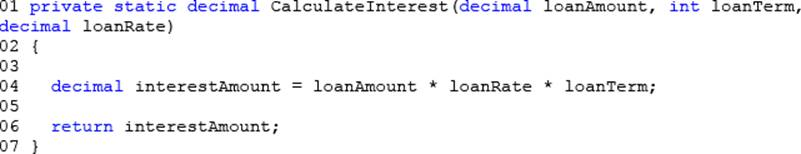# What should you do?

You are debugging an application that calculates loan interest.

The application includes the following code. (Line numbers are included for reference only.)You need to ensure that the debugger breaks execution within the CalculateInterest() method when the loanAmount variable is less than or equal to zero in all builds of the application.

What should you do?
A . Insert the following code segment at line 03: Trace.Assert(loanAmount > 0);
B . Insert the following code segment at line 03: Debug.Assert(loanAmount > 0);
C . Insert the following code segment at line 05: Debug.Write(loanAmount > 0);
D . Insert the following code segment at line 05: Trace.Write(loanAmount > 0);